##### Statistics: 1001 Practice Problems For Dummies (+ Free Online Practice)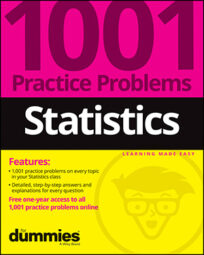The normal distribution is the most common distribution of all. Its values take on that familiar bell shape, with more values near the center and fewer as you move away. Solve the following problems about the definition of the normal distribution and what it looks like.

## Sample questions

1. What are properties of the normal distribution?

(A) It's symmetrical.

(B) Mean and median are the same.

(C) Most common values are near the mean; less common values are farther from it.

(D) Standard deviation marks the distance from the mean to the inflection point.

(E) All of the above.

The properties of the normal distribution are that it's symmetrical, mean and median are the same, the most common values are near the mean and less common values are farther from it, and the standard deviation marks the distance from the mean to the inflection point.

2. In a normal distribution, about what percent of the values lie within one standard deviation of the mean?

The empirical rule (also known as the 68-95-99.7 rule) says that about 68% of the values in a normal distribution are within one standard deviation of the mean.

3. In a normal distribution, about what percent of the values lie within two standard deviations of the mean?

The empirical rule (also known as the 68-95-99.7 rule) says that about 95% of the values in a normal distribution are within two standard deviations of the mean.

4. In a normal distribution, about what percent of the values lie within three standard deviations of the mean?

The empirical rule (also known as the 68-95-99.7 rule) says that about 99.7% of the values in a normal distribution are within three standard deviations of the mean.

5. What two parameters (pieces of information about the population) are needed to describe a normal distribution?

Answer: the mean and the standard deviation

You can re-create any normal distribution if you know two parameters: the mean and the standard deviation. The mean is the center of the bell-shaped picture, and the standard deviation is the distance from the mean to the inflection point (the place where the concavity of the curve changes on the graph).

6. For a normal distribution with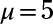and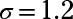34% of the values lie between 5 and what number? (Assume that the number is above the mean.)

You want to find a value of X where 34% of the values lie between the mean (5) and x (and x is in the right side of the mean). First, note that the normal distribution has a total probability of 100%, and each half takes up 50%. You'll use the 50% idea to do this problem.

Because this is a normal distribution, according to the empirical rule, about 68% of values are within one standard deviation from the mean on either side. That means that about 34% are within one standard deviation above the mean.

If x is one standard deviation above its mean, x equals the mean (5) plus 1 times the standard deviation (1.2): x = 5 + 1(1.2) = 6.2.

7. For a normal distribution withandabout 2.5% of the values lie above what value? (Assume that the number is above the mean.)

You want to find a value of X where 2.5% of the values are greater than x. First, note that the normal distribution has a total probability of 100%, and each half takes up 50%. You'll use this 50% idea to do this problem.

Because this is a normal distribution, according to the empirical rule, about 95% of values are within two standard deviations from the mean on either side.

That means that about 47.5% are within two standard deviations above the mean, and beyond that point, you have about (50 – 47.5) = 2.5% of the values. (Remember that the total percentage above the mean equals 50%.) The value of X where this occurs is the one that's two standard deviations above its mean.

If x is two standard deviations above its mean, x equals the mean (5) plus 2 times the standard deviation (1.2): x = 5 + 2(1.2) = 7.4.

8. What are the mean and standard deviation of the Z-distribution?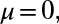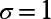The Z-distribution, also called the standard normal distribution, has a mean of 0 and a standard deviation of 1.

If you need more practice on this and other topics from your statistics course, visit 1,001 Statistics Practice Problems For Dummies to purchase online access to 1,001 statistics practice problems! We can help you track your performance, see where you need to study, and create customized problem sets to master your stats skills.